## How to Calculate and Solve for Length of Well Screen | Water Budget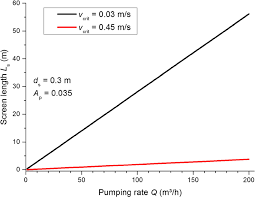The image above represents length of well screen.

To compute for length of well screen, three essential parameters are needed and these parameters are Maximum Expected Discharge Capacity of Well (Qo), Effective Open Area per Metre (Ao) and Entrance Velocity at the Screen (Ve).

The formula for calculating length of well screen:

L = Qo / AoVe

Where:

L = Length of Well Screen
Qo = Maximum Expected Discharge Capacity of Well
Ao = Effective Open Area per Metre
Ve = Entrance Velocity at the Screen

Let’s solve an example;
Find the length of well screen when the maximum expected discharge capacity of well is 12, the effective open area per metre is 24 and the entrance velocity at the screen is 18.

This implies that;

Qo = Maximum Expected Discharge Capacity of Well
Ao = Effective Open Area per Metre
Ve = Entrance Velocity at the Screen

L = Qo / AoVe
L = 12 / (24)(18)
L = 12 / 432
L = 0.027

Therefore, the length of well screen is 0.027.

Calculating the Maximum Expected Discharge Capacity of Well when the Length of Well Screen, the Effective Open Area per Metre and the Entrance Velocity at the Screen is Given.

Qo = L x (AoVe)

Where;

Qo = Maximum Expected Discharge Capacity of Well
L = Length of Well Screen
Ao = Effective Open Area per Metre
Ve = Entrance Velocity at the Screen

Let’s solve an example;
Find the maximum expected discharge capacity of well with a length of well screen as 21, effective open area per metre as 10 and entrance velocity at the screen as 5.

This implies that;

L = Length of Well Screen = 21
Ao = Effective Open Area per Metre = 10
Ve = Entrance Velocity at the Screen = 5

Qo = L x (AoVe)
Qo = 21 x (10 x 5)
Qo = 21 x 50
Qo = 1050

Therefore, the maximum expected discharge capacity of well is 1050.

## How to Calculate and Solve for Hydrostatic Pressure of a Dam | Water Budget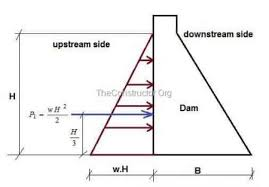The image above represents hydrostatic pressure of a dam.

To compute for hydrostatic pressure of a dam, two essential parameters are needed and these parameters are Specific Weight of Water (γ) and Depth of water (h).

The formula for calculating hydrostatic pressure of a dam:

Hn = γh² / 2

Where:

Hn = Hydrostatic Pressure of a Dam
γ = Specific Weight of Water
h = Depth of Water

Let’s solve an example;
Find the hydrostatic pressure of a dam when the specific weight of water is 10 and the depth of water is 5.

This implies that;

γ = Specific Weight of Water = 10
h = Depth of Water = 5

Hn = γh² / 2
Hn = (10)(5)² / 2
Hn = 10(25) / 2
Hn = 250 / 2
Hn = 125

Therefore, the hydrostatic pressure of the dam is 125.

Calculating the Specific Weight of Water when the Hydrostatic Pressure of the Dam and the Depth of Water is Given.

γ = Hn x 2 / h2

Where;

γ = Specific Weight of Water
Hn = Hydrostatic Pressure of a Dam
h = Depth of Water

Let’s solve an example;
Find the specific weight of water when the hydrostatic pressure of a dam is 30 and the depth of water is 6.

This implies that;

Hn = Hydrostatic Pressure of a Dam = 30
h = Depth of Water = 6

γ = Hn x 2 / h2
γ = 30 x 2 / 62
γ = 60 / 36
γ = 1.667

Therefore, the specific weight of water is 1.667.

## How to Calculate and Solve for Entrance Velocity | Darcy’s Law | Water Budget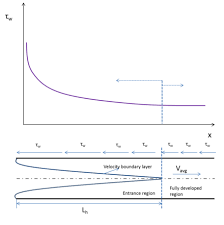The image above represents entrance velocity.

To compute for entrance velocity, one essential parameters are needed and these parameters are Hydraulic Conductivity (ki).

The formula for calculating entrance velocity:

Ve = ki

Where:

Ve = Entrance Velocity
ki = Hydraulic Conductivity

Let’s solve an example;
Find the entrance velocity when the hydraulic conductivity is 8.\

This implies that;

ki = Hydraulic Conductivity = 8

Ve = ki
Ve = 8

Therefore, the entrance velocity is 8.

## How to Calculate and Solve for Storage Co-efficient for a Confined Aquifer | Water Budget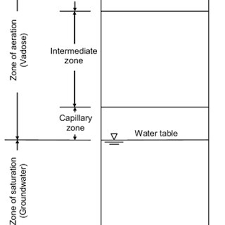The image above represents storage co-efficient for a confined aquifer.

To compute for storage co-efficient for a confined aquifer, five essential parameters are needed and these parameters are Unit Weight of Water (γw), Confined Aquifer Thickness (H), Compressibility of the Aquifer Material (α), Porosity of Aquifer Material (n) and Compressibility of Water (β).

The formula for calculating storage co-efficient for a confined aquifer:

S = γw(α + nβ)H

Where;

S = Storage Co-efficient for a Confined Aquifer
γw = Unit Weight of Water
H = Confined Aquifer Thickness
α = Compressibility of the Aquifer Material
n = Porosity of Aquifer Material
β = Compressibility of Water

Let’s solve an example;
Find the storage co-efficient for a confined aquifer when the unit weight of water is 8, confined aquifer thickness is 12, the compressibility of the aquifer material is 21, the porosity of aquifer material is 18 and the compressibiity of water is 14.

This implies that;

γw = Unit Weight of Water = 8
H = Confined Aquifer Thickness = 12
α = Compressibility of the Aquifer Material = 21
n = Porosity of Aquifer Material = 18
β = Compressibility of Water = 14

S = γw(α + nβ)H
S = 8(21 + 18(14))12
S = 8(21 + 252)12
S = 8(273)12
S = 26208

Therefore, the storage co-efficient for a confined aquifer is 26208.

Calculating the Unit Weight of Water when the Storage Co-efficient for a Confined Aquifer, the Confined Aquifer Thickness, the Compressibility of the Aquifer Material, the Porosity of Aquifer Material and the Compressibility of Water is Given.

γw = S / (a + nβ)H

Where;

γw = Unit Weight of Water
S = Storage Co-efficient for a Confined Aquifer
H = Confined Aquifer Thickness
α = Compressibility of the Aquifer Material
n = Porosity of Aquifer Material
β = Compressibility of Water

Let’s solve an example;
Find the unit weight of water when the storage co-efficient for a confined aquifer is 20, the confined aquifer thickness is 10, the compressibility of the aquifer material is 8, the porosity of aquifer material is 3 and the compressibility of water is 4.

This implies that;

S = Storage Co-efficient for a Confined Aquifer = 20
H = Confined Aquifer Thickness = 10
α = Compressibility of the Aquifer Material = 8
n = Porosity of Aquifer Material = 3
β = Compressibility of Water = 4

γw = S / (a + nβ)H
γw = 20 / (8 + (3 x 4))10
γw = 20 / (8 + 12)10
γw = 20 / (20)10
γw = 20 / 200
γw = 0.1

Therefore, the unit weight of water is 0.1.

## How to Calculate and Solve for Discharge Current | Unconfined Aquifer | Water Budget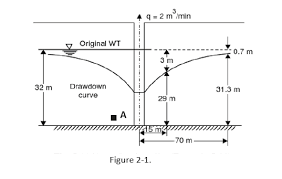The image above represents discharge current.

To compute for discharge current, five essential parameters are needed and these parameters are Hydraulic conductivity (k), Elevate from impermeable to surface (H), Depth of water in the well (hw), Radius of influence (R) and Radius of well (γw).

The formula for calculating discharge current:

Q = πk(H² – hw²) / In[R/γw]

Where:

Q = Discharge Current
k = Hydraulic Conductivity
H = Elevate from Impermeable to Surface
hw = Depth of Water in the Well

Let’s solve an example;
Find the discharge current when the hydraulic conductivity is 10,the elevate from impermeable to surface is 4, the depth of water in the well is 20, the radius of influence is 5 and the radius of well is 10.

This implies that;

k = Hydraulic Conductivity = 10
H = Elevate from Impermeable to Surface = 4
hw = Depth of Water in the Well = 20
R = Radius of Influence = 5
γw = Radius of Well = 10

Q = πk(H² – hw²) / In[R/γw]
Q = π(10)[(4)² – (20)²] / In[5/10]
Q = 31.415[16 – 400] / In[0.5]
Q = 31.415[-384] / -0.693
Q = -12063.7 / -0.69
Q = 17404.26

Therefore, the discharge current is 17404.26.

## How to Calculate and Solve for Rate | Salt Trace Method | Water Budget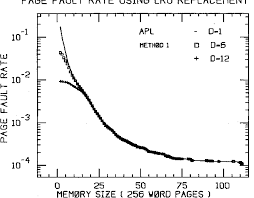The image above represents rate.

To compute for rate, three essential parameters are needed and these parameters are Rate of injection for salt solution (q), Concentration of salt solution (c1) and Concentration of river downstream (c2).

The formula for calculating rate:

Q = q(c1 – c2)

Where:

Q = Rate
q = Rate of Injection for Salt Solution or Trace
c1 = Concentration of Salt Solution or Trace
c2 = Concentration of River Downstream

Let’s solve an example;
Find the rate when the rate of injection for salt solution is 12, the concentration of salt solution is 20 and the concentration of river downstream is 18.

This implies that;

q = Rate of Injection for Salt Solution or Trace = 12
c1 = Concentration of Salt Solution or Trace = 20
c2 = Concentration of River Downstream = 18

Q = q(c1 – c2)
Q = 12(20 – 18)
Q = 12(2)
Q = 24

Therefore, the rate is 24.

Calculating the Rate of Injection for Salt Solution when the Rate, the Concentration of Salt Solution and the Concentration of River Downstream is Given.

q = Q / (c1 – c2)

Where;

q = Rate of Injection for Salt Solution or Trace
Q = Rate
c1 = Concentration of Salt Solution or Trace
c2 = Concentration of River Downstream

Let’s solve an example;
Find the rate of injection for salt solution when the rate is 40, the concentration of salt solution is 32 and the concentration of river downstream is 14.

This implies that;

Q = Rate = 40
c1 = Concentration of Salt Solution or Trace = 32
c2 = Concentration of River Downstream = 14

q = Q / (c1 – c2)
q = 40 / (32 – 14)
q = 40 / 18
q = 2.2

Therefore, the rate of injection for salt solution is 2.2.

## How to Calculate and Solve for Discharge | Chezy’s Equation | Water Budget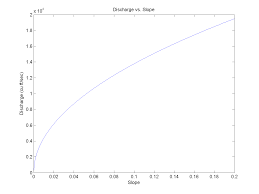The image above represents discharge | Chezy’s Equation.

To compute for discharge, four essential parameters are needed and these parameters are Area (A), Chezy’s Constant (C), Hydraulic Radius (R) and Slope (S).

The formula for calculating discharge:

Q = AC√(RS)

Where;

Q = Discharge
A = Area
C = Chezy’s Constant
S = Slope

Let’s solve an example;
Find the discharge when the area is 25, the chezy’s constant is 16, the hydraulic radius is 9 and the slope is 21.

This implies that;

A = Area = 25
C = Chezy’s Constant = 16
R = Hydraulic Radius = 9
S = Slope = 21

Q = AC√(RS)
Q = (25)(16)√((9)(21))
Q = (25)(16)√(189)
Q = (25)(16)(13.74)
Q = 5499.09

Therefore, the discharge according to Chezy’s equation is 5499.09.

Calculating the Area when the Discharge, the Chezy’s Constant, the Hydraulic Radius and the Slope is Given.

A = Q / C √RS

Where;

A = Area
Q = Discharge
C = Chezy’s Constant
S = Slope

Let’s solve an example;
Find the area when the discharge is 40, the chezy’s constant is 10, the hydraulic radius is 5 and the slope is 9.

This implies that;

Q = Discharge = 40
C = Chezy’s Constant = 10
R = Hydraulic Radius = 5
S = Slope = 9

A = Q / C √RS
A = 40 / 10 √(5)(9)
A = 40 / 10 √45
A = 40 / 10 (6.708)
A = 40 / 67.08
A = 0.596

Therefore, the area is 0.596.

## How to Calculate and Solve for Discharge | Slope Area Method | Water Budget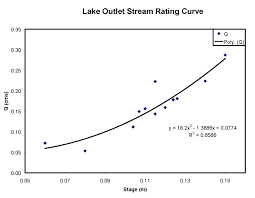The image above represents discharge | slope area method.

To compute for discharge | slope area method, four essential parameters are needed and these parameters are Manning’s Co-efficient (N), Area (A), Hydraulic Radius (R) and Slope (S).

The formula for calculating discharge | slope area method:

Q = (A / N) R2/3 S1/2

Where;

Q = Discharge
N = Manning’s Co-efficient
A = Area
S = Slope

Let’s solve an example;
Find the discharge when the manning’s co-efficient is 4, the area is 30, the hydraulic radius is 15 and the slope is 22.

This implies that;

N = Manning’s Co-efficient = 4
A = Area = 30
R = Hydraulic Radius = 15
S = Slope = 22

Q = (A / N) R2/3 S1/2
Q = (30 / 4) (15)2/3 (22)1/2
Q = (7.5) (6.08) (4.69)
Q = 213.96

Therefore, the discharge is 213.96.

## How to Calculate and Solve for Structure Capacity | Water Budget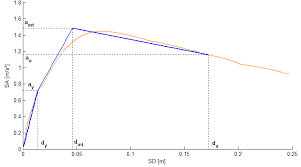The image above represents structure capacity.

To compute for structure capacity, three essential parameters are needed and these parameters are Entrance Factor (C), Crest Length (L) and Depth of Flow (H).

The formula for calculating the structure capacity:

Q = CLH1.5

Where:

Q = Structure Capacity
C = Entrance Factor
L = Crest Length
H = Depth of Flow

Let’s solve an example;
Find the structure capacity when the entrance factor is 6, the crest length is 10 and the depth of flow is 12.

This implies that;

C = Entrance Factor = 6
L = Crest Length = 10
H = Depth of Flow = 12

Q = CLH1.5
Q = (6)(10)(12)1.5
Q = (6)(10)(41.56)
Q = 2494.15

Therefore, the structure capacity is 2494.15.

Calculating the Entrance Factor when the Structure Capacity, the Crest Length and the Depth of Flow is Given.

C = Q / LH1.5

Where;

C = Entrance Factor
Q = Structure Capacity
L = Crest Length
H = Depth of Flow

Let’s solve an example;
Find the entrance factor when the structure capacity is 20, the crest length is 8 and the depth of flow is 4.

This implies that;

Q = Structure Capacity = 20
L = Crest Length = 8
H = Depth of Flow = 4

C = Q / LH1.5
C = 20 / (8)(8)
C = 20 / 64
C = 0.3125

Therefore, the entrance factor is 0.3125.

## How to Calculate and Solve for Quantity of Soil Moved | Water Budget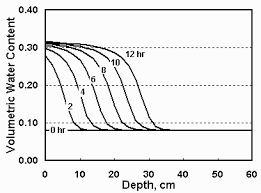The image above represents quantity of soil moved.

To compute for quantity of soil moved, two essential parameters are needed and these parameters are Excess Wind Velocity (vw) and Particle Diameter (dp).

The formula for calculating quantity of soil moved:

Q = vw³ . dp

Where;

Q = Quantity of Soil Moved
vw = Excess Wind Velocity
dp = Particle Diameter

Let’s solve an example;
Find the quantity of soil moved when the excess wind velocity is 12 and the particle diameter is 20.

This implies that;

vw = Excess Wind Velocity = 12
dp = Particle Diameter = 20

Q = vw³ . dp
Q = (12)³ . (20)
Q = (1728) . (20)
Q = 34560

Therefore, the quantity of soil moved is 34560.

Calculating the Excess Wind Velocity when the Quantity of Soil Moved and the Particle Diameter is Given.

vw³ = 3Q / dp

Where;

vw = Excess Wind Velocity
Q = Quantity of Soil Moved
dp = Particle Diameter

Let’s solve an example;
Find the excess wind velocity when the quantity of soil moved is 30 and the particle diameter is 10.

This implies that;

Q = Quantity of Soil Moved = 30
dp = Particle Diameter = 10

vw³ = 3Q / dp
vw³ = 330 / 10
vw³ = 3√3
vw³ = 1.442

Therefore, the excess wind velocity is 1.442.